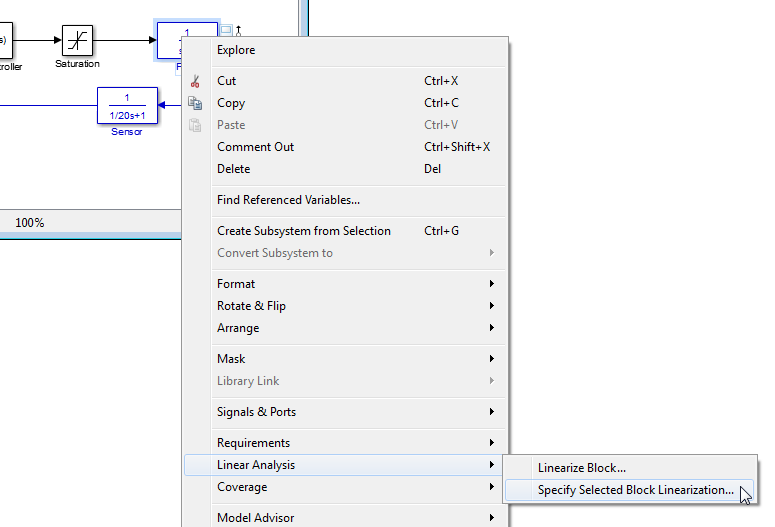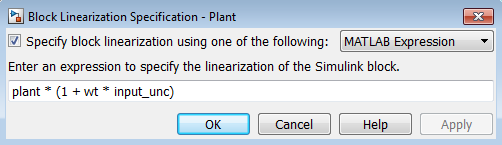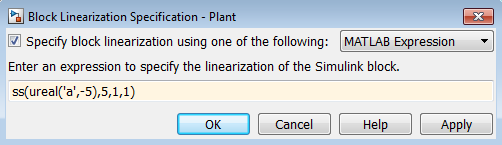## Compute Uncertain State-Space Models from Simulink Models

When you have the Simulink® Control Design™ software, you can compute an uncertain linearization, i.e., an uncertain state-space model (`uss`) combining the uncertain variables with linearized dynamics. Use the `uss` model to perform linear analysis and robust control design.

You can compute an uncertain linearization in one of the following ways:

### Obtain Uncertain State-Space Model from Simulink Model

To obtain an uncertain state-space model from a model that contains Uncertain State Space blocks, use the following steps:

### Note

If you do not have Uncertain State Space blocks in the model but still want to obtain an uncertain state-space model, see Specify Uncertain Linearization for Core or Custom Simulink Blocks.

1. (Prerequisite) Create or open the Simulink model.

2. (Prerequisite) In the Simulink model, specify the linearization input and output points using Simulink Control Design `getlinio` or `linio` commands. For more information, see Specify Portion of Model to Linearize (Simulink Control Design).

3. (Prerequisite) If you have not already done so, specify uncertainty in the Simulink model as described in Specify Uncertainty Using Uncertain State Space Blocks.

### Note

The software does not evaluate the uncertain variables during linearization. Thus, the value of the uncertainty does not affect the linearization.

4. Run `ulinearize` to compute an uncertain linearization. This command returns an `uss` model.

### Note

If you use the Simulink Control Design`linearize` command, the Uncertain State Space blocks linearize to their nominal value.

For more information on linearization and how to evaluate the results, see Linearization Basics (Simulink Control Design).

For an example of how to use the Simulink Control Design `linearize` command, see Linearization of Simulink Models with Uncertainty.

### Specify Uncertain Linearization for Core or Custom Simulink Blocks

In some cases, you cannot use Uncertain State Space blocks in the Simulink model because you share the model or generate code. You can still account for uncertainty in your linear analysis without specifying uncertainty using Uncertain State Space blocks. Robust Control Toolbox™ lets you specify a core or custom Simulink block to linearize to an uncertain variable. The linearization produces an uncertain state-space `uss` model. The specified uncertainty associates only with the block and does not affect the model simulation. For more information, see Specify Linear System for Block Linearization Using MATLAB Expression (Simulink Control Design).

### Note

If you have Uncertain State Space blocks in the model and want to obtain an uncertain state-space model, see Obtain Uncertain State-Space Model from Simulink Model.

To specify blocks to linearize to uncertain variables and obtain an uncertain state-space model:

1. (Prerequisites) Create or open the Simulink model. Specify linearization input and output points using the Simulink Control Design `getlinio` or `linio` commands.

For this example, you can open the model `rct_ulinearize_builtin`.

2. Specify a block to linearize to an uncertain variable:

1. Right-click the block and select Linear Analysis > Specify Selected Block Linearization.This action opens the Block Linearization Specification dialog box.2. In the Block Linearization Specification dialog box, select the Specify block linearization using one of the following: check box. Selecting this check box lets you to specify an uncertain variable for linearization.

This check box defaults to `MATLAB Expression` in the drop-down menu. This option lets you specify the block to linearize to an uncertain variable using a MATLAB® expression containing Robust Control Toolbox functions. To learn more about the options, see Specify Linear System for Block Linearization Using MATLAB Expression (Simulink Control Design).

3. In the Enter an expression to specify the linearization of the Simulink block field, enter an expression, which must evaluate to an uncertain variable or uncertain model, such as `ureal`, `umat`, `ultidyn` or `uss`.4. Click OK to save the changes.

### Note

You can also specify a block to linearize to an uncertain variable at the command line. For an example, see Linearize Block to Uncertain Model.

3. Run the `linearize` command to compute an uncertain linearization. This command returns an `uss` model.

For more information on linearization and how to validate linearization results, see Linearization Basics (Simulink Control Design).

For an example of how to use the `linearize` command to compute an uncertain linearization, see Linearization of Simulink Models with Uncertainty.

### Using Uncertain Linearization for Analysis or Control Design

After computing an uncertain linearization, you can perform any analysis or design tasks you would perform on any linear model, including: